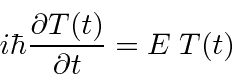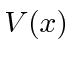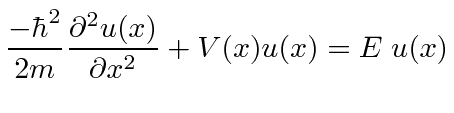## The Time Independent Schrödinger Equation

Second order differential equations, like the Schrödinger Equation, can be solved by separation of variables. These separated solutions can then be used to solve the problem in general.

Assume that we can factorize the solution between time and space.Plug this into the Schrödinger Equation.Put everything that depends onon the left and everything that depends onon the right.Since we have a function of onlyset equal to a function of only, they both must equal a constant. In the equation above, we call the constant, (with some knowledge of the outcome). We now have an equation inset equal to a constantwhich has a simple general solution,and an equation inset equal to a constantwhich depends on the problem to be solved (through).

Theequation is often called the Time Independent Schrödinger Equation.Here,is a constant. The full time dependent solution is.* Example: Solve the Schrödinger equation for a constant potential.*

Jim Branson 2013-04-22